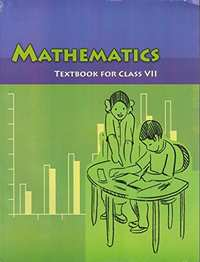# NCERT solutions for Class 7 Maths chapter 10 - Practical Geometry [Latest edition]

#### Chapters## Chapter 10: Practical Geometry

Exercise 10.1Exercise 10.2Exercise 10.3Exercise 10.4Exercise 10.5
Exercise 10.1 [Page 196]

### NCERT solutions for Class 7 Maths Chapter 10 Practical GeometryExercise 10.1 [Page 196]

Exercise 10.1 | Q 1 | Page 196

Draw a line, say AB, take a point C outside it. Through C, draw a line parallel to AB using ruler and compasses only

Exercise 10.1 | Q 2 | Page 196

Draw a line l. Draw a perpendicular to l at any point on l. On this perpendicular choose a point X, 4 cm away from l. Through X, draw a line m parallel to l.

Exercise 10.1 | Q 3 | Page 196

Let l be a line and P be a point not on l. Through P, draw a line m parallel to l. Now join P to any point Q on l. Choose any other point R on m. Through R, draw a line parallel to PQ. Let this meet l at S. What shape do the two sets of parallel lines enclose?

Exercise 10.2 [Page 199]

### NCERT solutions for Class 7 Maths Chapter 10 Practical GeometryExercise 10.2 [Page 199]

Exercise 10.2 | Q 1 | Page 199

Construct ΔXYZ in which XY = 4.5 cm, YZ = 5 cm and ZX = 6 cm.

Exercise 10.2 | Q 2 | Page 199

Construct an equilateral triangle of side 5.5 cm.

Exercise 10.2 | Q 3 | Page 199

Draw ΔPQR with PQ = 4 cm, QR = 3.5 cm and PR = 4 cm. What type of triangle is this?

Exercise 10.2 | Q 4 | Page 199

Construct ΔABC such that AB = 2.5 cm, BC = 6 cm and AC = 6.5 cm. Measure ∠B.

Exercise 10.3 [Page 200]

### NCERT solutions for Class 7 Maths Chapter 10 Practical GeometryExercise 10.3 [Page 200]

Exercise 10.3 | Q 1 | Page 200

Construct ΔDEF such that DE = 5 cm, DF = 3 cm and m∠EDF = 90°.

Exercise 10.3 | Q 2 | Page 200

Construct an isosceles triangle in which the lengths of each of its equal sides is 6.5 cm and the angle between them is 110°.

Exercise 10.3 | Q 3 | Page 200

Construct ΔABC with BC = 7.5 cm, AC = 5 cm and m∠C = 60°.

Exercise 10.4 [Page 202]

### NCERT solutions for Class 7 Maths Chapter 10 Practical GeometryExercise 10.4 [Page 202]

Exercise 10.4 | Q 1 | Page 202

Construct ΔABC, given m∠A = 60°, m∠B = 30° and AB = 5.8 cm.

Exercise 10.4 | Q 2 | Page 202

Construct ΔPQR if PQ = 5 cm, m∠PQR = 105° and m∠QRP = 40°. (Hint: Recall angle sum property of a triangle).

Exercise 10.4 | Q 3 | Page 202

Examine whether you can construct ΔDEF such that EF = 7.2 cm, m∠E = 110° and m∠F = 80°. Justify your answer.

Exercise 10.5 [Page 203]

### NCERT solutions for Class 7 Maths Chapter 10 Practical GeometryExercise 10.5 [Page 203]

Exercise 10.5 | Q 1 | Page 203

Construct the right angled ΔPQR, where m∠Q = 90°, QR = 8 cm and PR = 10 cm.

Exercise 10.5 | Q 2 | Page 203

Construct a right-angled triangle whose hypotenuse is 6 cm long and one of the legs is 4 cm long

Exercise 10.5 | Q 3 | Page 203

Construct an isosceles right-angled triangle ABC, where, m∠ACB = 90° and AC = 6 cm.

## Chapter 10: Practical Geometry

Exercise 10.1Exercise 10.2Exercise 10.3Exercise 10.4Exercise 10.5## NCERT solutions for Class 7 Maths chapter 10 - Practical Geometry

NCERT solutions for Class 7 Maths chapter 10 (Practical Geometry) include all questions with solution and detail explanation. This will clear students doubts about any question and improve application skills while preparing for board exams. The detailed, step-by-step solutions will help you understand the concepts better and clear your confusions, if any. Shaalaa.com has the CBSE Class 7 Maths solutions in a manner that help students grasp basic concepts better and faster.

Further, we at Shaalaa.com provide such solutions so that students can prepare for written exams. NCERT textbook solutions can be a core help for self-study and acts as a perfect self-help guidance for students.

Concepts covered in Class 7 Maths chapter 10 Practical Geometry are Construction of a Line Parallel to a Given Line, Through a Point Not on the Line, Construction of Triangles, Constructing a Triangle When the Length of Its Three Sides Are Known (SSS Criterion), Constructing a Triangle When the Lengths of Two Sides and the Measure of the Angle Between Them Are Known. (SAS Criterion), Constructing a Triangle When the Measures of Two of Its Angles and the Length of the Side Included Between Them is Given. (ASA Criterion), Constructing a Right-angled Triangle When the Length of One Leg and Its Hypotenuse Are Given (RHS Criterion).

Using NCERT Class 7 solutions Practical Geometry exercise by students are an easy way to prepare for the exams, as they involve solutions arranged chapter-wise also page wise. The questions involved in NCERT Solutions are important questions that can be asked in the final exam. Maximum students of CBSE Class 7 prefer NCERT Textbook Solutions to score more in exam.

Get the free view of chapter 10 Practical Geometry Class 7 extra questions for Class 7 Maths and can use Shaalaa.com to keep it handy for your exam preparation